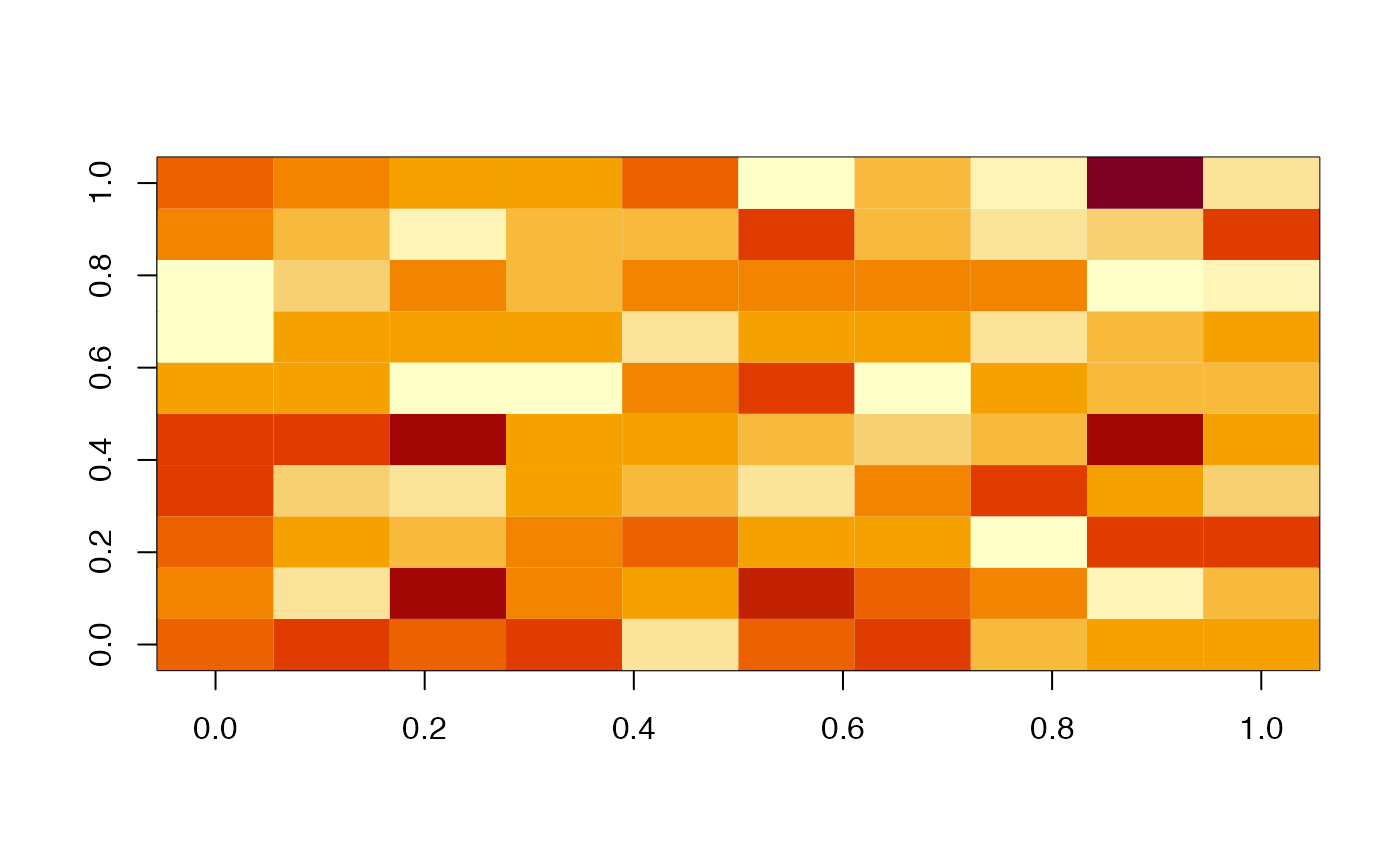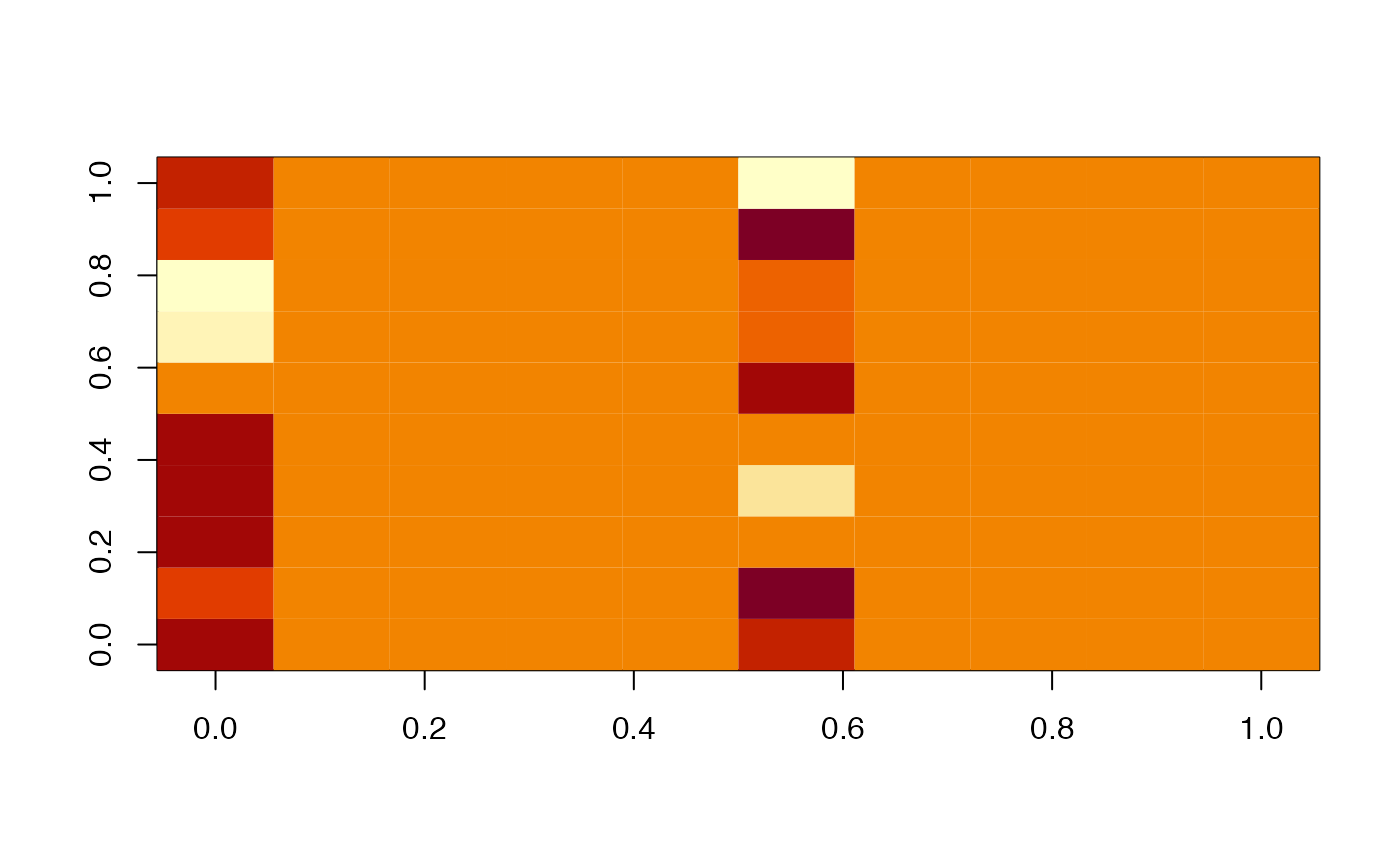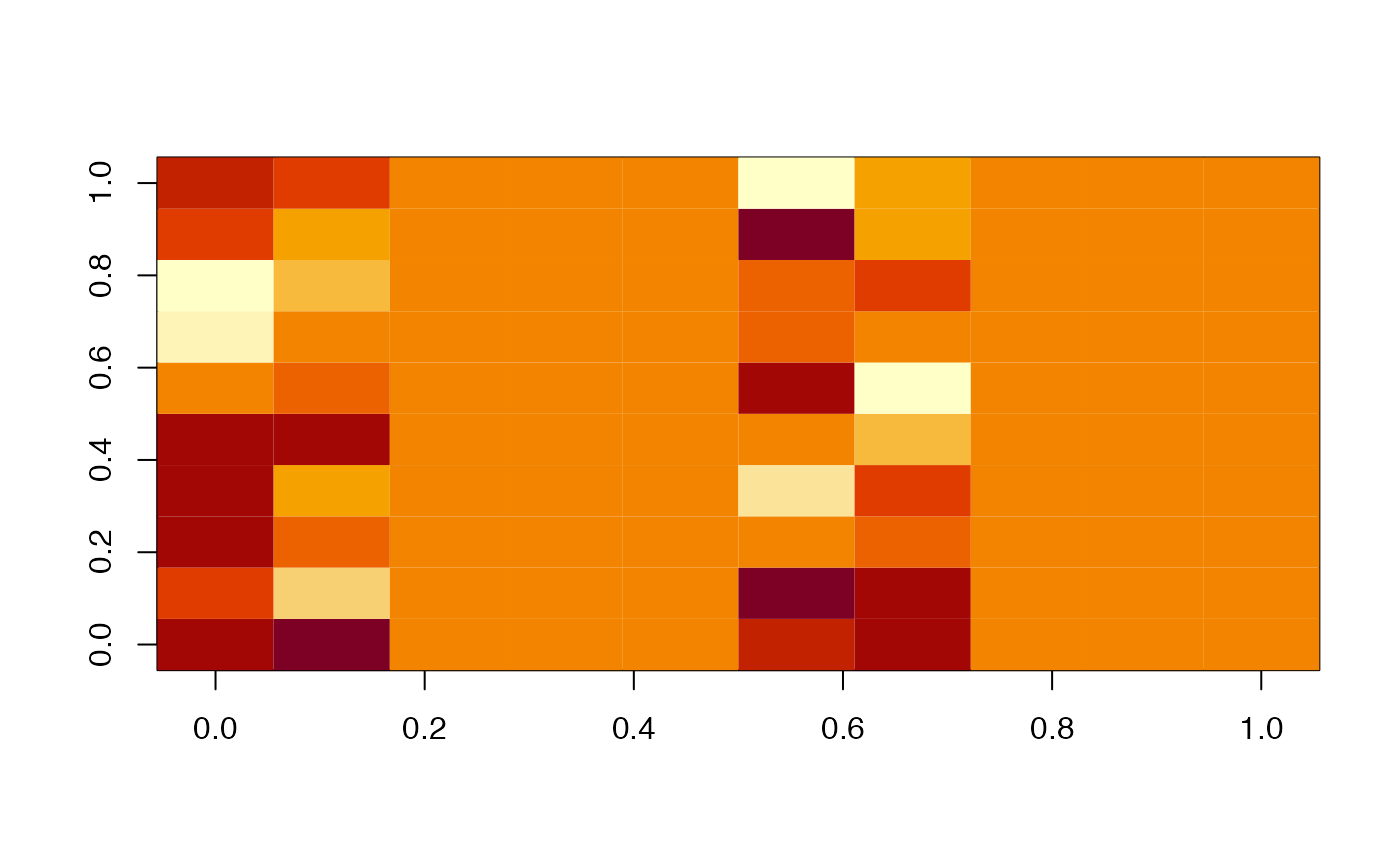Mask an object, typically to produce a copy with some values zeroed out

mask(x, ...)

# S3 method for im3d
mask(x, mask, levels = NULL, rval = c("im3d", "values"), invert = FALSE, ...)

## Arguments

x

...

An im3d object, an array or a vector with dimensions compatible with x.

levels

Optional numeric vector of pixel values or character vector defining named materials.

rval

Whether to return an im3d object based on x or just the values from x matching the mask.

invert

Whether to invert the voxel selection (default FALSE)

## Value

an object with attributes matching x and elements with value as.vector(TRUE, mode=mode) i.e. TRUE, 1, 0x01 and as.vector(FALSE, mode=mode) i.e. FALSE, 0, 0x00 as appropriate. A copy of x with

## Details

Note that mask.im3d passes ... arguments on to im3d

Other im3d: as.im3d(), boundingbox(), im3d-coords, im3d-io, im3d(), imexpand.grid(), imslice(), is.im3d(), origin(), projection(), threshold(), unmask(), voxdims()

## Examples

x=im3d(array(rnorm(1000),dim=c(10,10,10)), BoundingBox=c(20,200,100,200,200,300))
m=array(1:5,dim=c(10,10,10))
image(x[,,1])